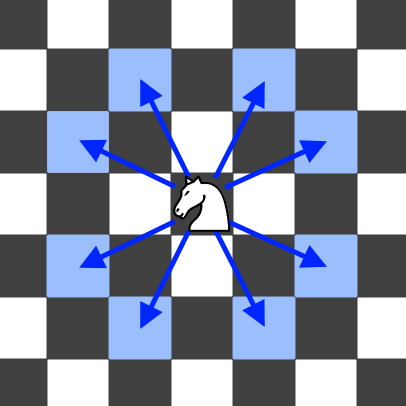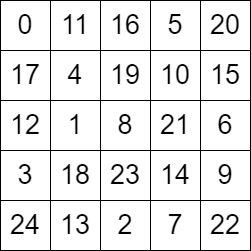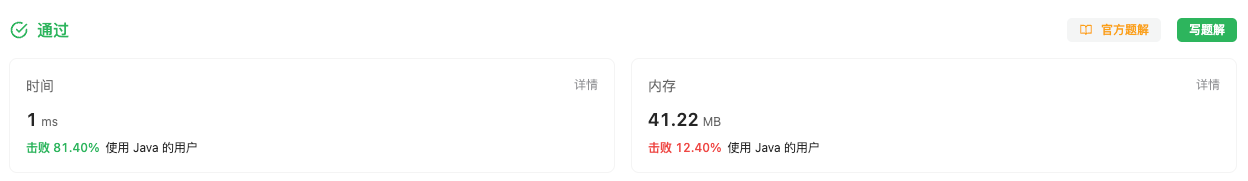#### 2596. 检查骑士巡视方案

###### 来源: 每日一题 2023.09.13``````输入：grid = [[0,11,16,5,20],[17,4,19,10,15],[12,1,8,21,6],[3,18,23,14,9],[24,13,2,7,22]]

````````````输入：grid = [[0,3,6],[5,8,1],[2,7,4]]

``````

• `n == grid.length == grid[i].length`

• `3 <= n <= 7`

• `0 <= grid[row][col] < n * n`

• `grid 中的所有整数 互不相同`

``````class Solution {
public boolean checkValidGrid(int[][] grid) {

}
}
``````

#### 分析与题解

• HashMap + 向量的距离

这个题目的解题关键是怎么知道, 我们可以去往下一个点? 这个很简单, 我们判断一下当前节点和下一个节点是一个 `日` 字形状即可. 那么表现形式是什么呢? 也就是 `x 方向的距离``y 方向的距离` 乘积为 `2`

知道上面的条件, 我们首先处理边界条件, 也就是说我们必须从 左上角 开始巡逻.

``````if (grid != 0) {
return false;
}
``````

然后查找所有点的位置, 并且进行存储.

``````// 先查找所有点的位置
Map<Integer, Integer[]> cache = new HashMap<>();
for(int i = 0; i < grid.length; i++) {
int[] group = grid[i];
for(int j = 0; j < group.length; j++) {
cache.put(grid[i][j], new Integer[]{i, j});
}
}
``````

构建好完整的HashMap , 我么就遍历所有的节点. 判断当前节点和下一个节点是否满足距离要求. 如果不满足就直接 `return false;`

``````int count =  cache.keySet().size() - 1;
for(int i = 0; i < count; i++) {
Integer[] firstGroup = cache.get(i);
Integer[] secondGroup = cache.get(i + 1);
int x = Math.abs(firstGroup - secondGroup);
int y = Math.abs(firstGroup - secondGroup);
// 判断向量之间的距离即可
if (x * y != 2) {
return false;
}
}
``````

如果查找到最后仍然符合条件. 那也就是整个矩阵的点都能巡逻到, 符合题意, 返回 `true` 即可.

接下来, 我们一起看下完整的解题过程.

``````class Solution {
public boolean checkValidGrid(int[][] grid) {
if (grid != 0) {
return false;
}
// 先查找所有点的位置
Map<Integer, Integer[]> cache = new HashMap<>();
for(int i = 0; i < grid.length; i++) {
int[] group = grid[i];
for(int j = 0; j < group.length; j++) {
cache.put(grid[i][j], new Integer[]{i, j});
}
}
// 遍历查找点是否合适 从 0 查到 cache.keySet().size() - 1 - 1, 最后一个点不用查
int count =  cache.keySet().size() - 1;
for(int i = 0; i < count; i++) {
Integer[] firstGroup = cache.get(i);
Integer[] secondGroup = cache.get(i + 1);
int x = Math.abs(firstGroup - secondGroup);
int y = Math.abs(firstGroup - secondGroup);
// 判断向量之间的距离即可
if (x * y != 2) {
return false;
}
}
return true;
}
}
``````

复杂度分析:

• 时间复杂度: O(n² + n), 需要遍历矩阵,所以时间复杂度为 `n²`, 然后再遍历一遍HashMap.
• 空间复杂度: O(n²)，HashMap的空间开辟与矩阵的空间大小成线性关系.

结果如下所示.IT界无底坑洞栋主 欢迎加Q骚扰:676758285